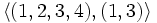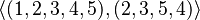# Subgroup structure of symmetric group:S5

TAKE A QUIZ ON THIS TOPIC and test the quality of your understanding of it
View subgroup structure of particular groups | View other specific information about symmetric group:S5

The symmetric group of degree five has many subgroups. We'll take the five letters as$\{ 1,2,3,4,5\}$. The group has order 120.

Note that since$S_5$ is a complete group, every automorphism of it is inner, so the classification of subgroups upto conjugacy is the same as the classification of subgroups upto automorphism. In other words, every subgroup is an automorph-conjugate subgroup.

## Tables for quick information

FACTS TO CHECK AGAINST FOR SUBGROUP STRUCTURE: (finite group)
Lagrange's theorem (order of subgroup times index of subgroup equals order of whole group, so both divide it), |order of quotient group divides order of group (and equals index of corresponding normal subgroup)
Sylow subgroups exist, Sylow implies order-dominating, congruence condition on Sylow numbers|congruence condition on number of subgroups of given prime power order
normal Hall implies permutably complemented, Hall retract implies order-conjugate

### Quick summary

Item Value
Number of subgroups 156
Compared with$S_n, n = 1,2,3,4,5,6,7,\dots$: 1,2,6,30,156,1455,11300, 151221
Number of conjugacy classes of subgroups 19
Compared with$S_n$,$n = 1,2,3,4,5,6,7,\dots$: 1,2,4,11,19,56,96,296,554,1593
Number of automorphism classes of subgroups 19
Compared with$S_n$,$n = 1,2,3,4,5,6,7,\dots$: 1,2,4,11,19,37,96,296,554,1593
Isomorphism classes of Sylow subgroups and the corresponding Sylow numbers and fusion systems 2-Sylow: dihedral group:D8 (order 8), Sylow number is 15, fusion system is non-inner non-simple fusion system for dihedral group:D8
3-Sylow: cyclic group:Z3, Sylow number is 10, fusion system is non-inner fusion system for cyclic group:Z3
5-Sylow: Z5 in S5, Sylow number is 6, fusion system is universal fusion system for cyclic group:Z5
Hall subgroups$\{ 2,3 \}$-Hall subgroup: S4 in S5 (order 24)
No$\{ 2,5 \}$-Hall subgroup or$\{ 3,5 \}$-Hall subgroup
maximal subgroups maximal subgroups have orders 12 (direct product of S3 and S2 in S5), 20 (GA(1,5) in S5), 24 (S4 in S5), 60 (A5 in S5)
normal subgroups There are three normal subgroups: the whole group, A5 in S5, and the trivial subgroup.

### Table classifying subgroups up to automorphisms

Note that the only normal subgroups are the trivial subgroup, the whole group, and A5 in S5, so we do not waste a column on specifying whether the subgroup is normal and on the quotient group.

TABLE SORTING AND INTERPRETATION: Note that the subgroups in the table below are sorted based on the powers of the prime divisors of the order, first covering the smallest prime in ascending order of powers, then powers of the next prime, then products of powers of the first two primes, then the third prime, then products of powers of the first and third, second and third, and all three primes. The rationale is to cluster together subgroups with similar prime powers in their order. The subgroups are not sorted by the magnitude of the order. To sort that way, click the sorting button for the order column. Similarly you can sort by index or by number of subgroups of the automorphism class.
Automorphism class of subgroups Representative subgroup (full list if small, generating set if large) Isomorphism class Order of subgroups Index of subgroups Number of conjugacy classes (= 1 iff automorph-conjugate subgroup) Size of each conjugacy class (= 1 iff normal subgroup) Total number of subgroups (= 1 iff characteristic subgroup) Note
trivial subgroup$()$ trivial group 1 120 1 1 1 trivial
S2 in S5$\{ (), (1,2) \}$ cyclic group:Z2 2 60 1 10 10
subgroup generated by double transposition in S5$\{ (), (1,2)(3,4) \}$ cyclic group:Z2 2 60 1 15 15
subgroup generated by pair of disjoint transpositions in S5$\{ (), (1,2), (3,4), (1,2)(3,4) \}$ Klein four-group 4 30 1 15 15
subgroup generated by double transpositions on four elements in S5$\{ (), (1,2)(3,4), (1,3)(2,4), (1,4)(2,3) \}$ Klein four-group 4 30 1 5 5
Z4 in S5$\{ (), (1,2,3,4), (1,3)(2,4), (1,4,3,2) \}$ cyclic group:Z4 4 30 1 15 15
D8 in S5$\langle (1,2,3,4), (1,3) \rangle$ dihedral group:D8 8 15 1 15 15 2-Sylow
Z3 in S5$\{ (), (1,2,3), (1,3,2) \}$ cyclic group:Z3 3 40 1 10 10 3-Sylow
Z6 in S5$\langle (1,2,3), (4,5) \rangle$ cyclic group:Z6 6 20 1 10 10
S3 in S5$\langle (1,2,3), (1,2) \rangle$ symmetric group:S3 6 20 1 10 10
twisted S3 in S5$\langle (1,2,3), (1,2)(4,5) \rangle$ symmetric group:S3 6 20 1 10 10
direct product of S3 and S2 in S5$\langle (1,2,3), (1,2), (4,5) \rangle$ direct product of S3 and Z2 12 10 1 10 10 3-Sylow normalizer
A4 in S5$\langle (1,2)(3,4), (1,2,3) \rangle$ alternating group:A4 12 10 1 5 5
S4 in S5$\langle (1,2,3,4), (1,2) \rangle$ symmetric group:S4 24 5 1 5 5 (2,3)-Hall
Z5 in S5$\langle (1,2,3,4,5) \rangle$ cyclic group:Z5 5 24 1 6 6 5-Sylow
D10 in S5$\langle (1,2,3,4,5), (2,5)(3,4) \rangle$ dihedral group:D10 10 12 1 6 6
GA(1,5) in S5$\langle (1,2,3,4,5), (2,3,5,4) \rangle$ general affine group:GA(1,5) 20 6 1 6 6
A5 in S5$\langle (1,2,3,4,5), (1,2,3)\rangle$ alternating group:A5 60 2 1 1 1 only proper nontrivial normal subgroup, quotient is cyclic group:Z2
whole group$\langle (1,2,3,4,5), (1,2) \rangle$ symmetric group:S5 120 1 1 1 1
Total (19 rows) -- -- -- -- 19 -- 156 --

### Table classifying isomorphism types of subgroups

Group name Order Second part of GAP ID (first part is order) Occurrences as subgroup Conjugacy classes of occurrence as subgroup Automorphism classes of occurrence as subgroup Occurrences as normal subgroup Occurrences as characteristic subgroup
trivial group 1 1 1 1 1 1 1
cyclic group:Z2 2 1 25 2 2 0 0
cyclic group:Z3 3 1 10 1 1 0 0
cyclic group:Z4 4 1 15 1 1 0 0
Klein four-group 4 2 20 2 2 0 0
cyclic group:Z5 5 1 6 1 1 0 0
symmetric group:S3 6 1 20 2 2 0 0
cyclic group:Z6 6 2 10 1 1 0 0
dihedral group:D8 8 3 15 1 1 0 0
dihedral group:D10 10 1 6 1 1 0 0
alternating group:A4 12 3 5 1 1 0 0
direct product of S3 and Z2 12 4 10 1 1 0 0
general affine group:GA(1,5) 20 3 6 1 1 0 0
symmetric group:S4 24 12 5 1 1 0 0
alternating group:A5 60 5 1 1 1 1 1
symmetric group:S5 120 34 1 1 1 1 1
Total -- -- 156 19 19 3 3

### Table listing number of subgroups by order

Note that these orders satisfy the congruence condition on number of subgroups of given prime power order: the number of subgroups of order$p^r$ is congruent to$1$ modulo$p$.

Group order Occurrences as subgroup Conjugacy classes of occurrence as subgroup Automorphism classes of occurrence as subgroup Occurrences as normal subgroup Occurrences as characteristic subgroup
1 1 1 1 1 1
2 25 2 2 0 0
3 10 1 1 0 0
4 35 3 3 0 0
5 6 1 1 0 0
6 30 3 3 0 0
8 15 1 1 0 0
10 6 1 1 0 0
12 15 2 2 0 0
20 6 1 1 0 0
24 5 1 1 0 0
60 1 1 1 1 1
120 1 1 1 1 1
Total 156 19 19 3 3